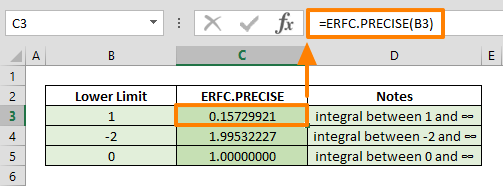The Excel ERFC.PRECISE (ERFC PRECISE) function is an Engineering formula that calculates and returns the complementary error function, integrated between a specified lower limit and infinity. This function has been introduced Excel 2010, and is not available in earlier versions. However, the ERFC.PRECISE function is similar to the ERFC function, which is available in earlier versions of Excel. In this guide, we’re going to show you how to use a variation of the ERFC functions, the ERFC.PRECISE function, and also go over some tips.

## Supported versions

• Excel 2010 and later

ERFC.PRECISE(x)

## Arguments

 x The lower bound of the integral.

## Example

The ERFC.PRECISE (ERFC PRECISE) function, just like the other ERFC function, only requires a single argument, x, as an input. The x value argument represents the lower bound for integrating the complementary error function (also known as the Gauss error function). The ERFC.PRECISE function can take negative numbers as an input for Excel versions 2010 and later. Below are some examples showing its use.

=ERFC.PRECISE(1) integrates between 1 and ∞ (infinity)## Tips

• Related Excel functions:
• Since the Excel 2010 version, ERFC function works exactly the same as the ERFC.PRECISE function. The precise tag is added to provide consistency between the function names.
• ERF function (the error function) integrates between a lower limit and upper limit.
• ERF.PRECISE function returns the error function between 0 and the entered argument.